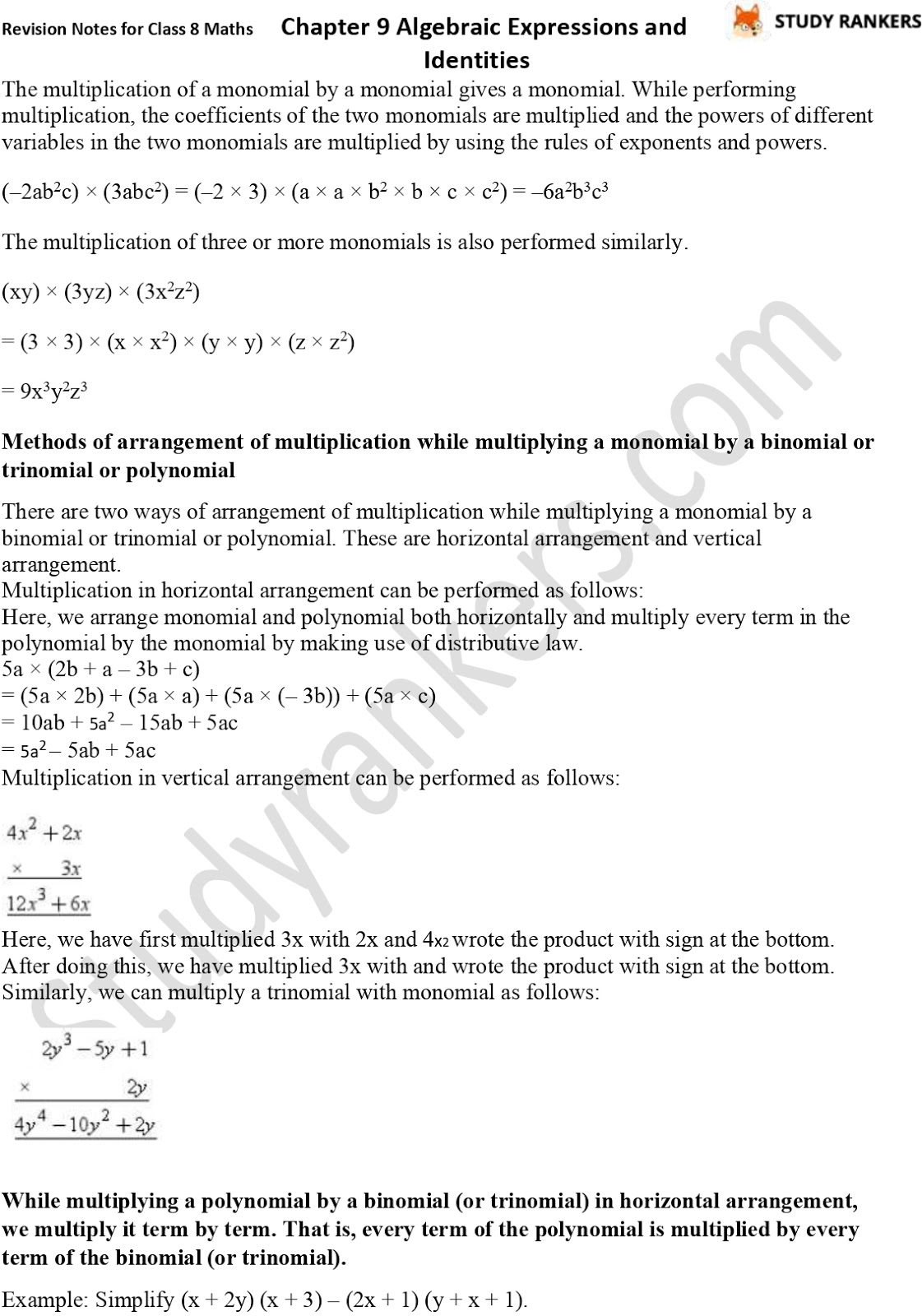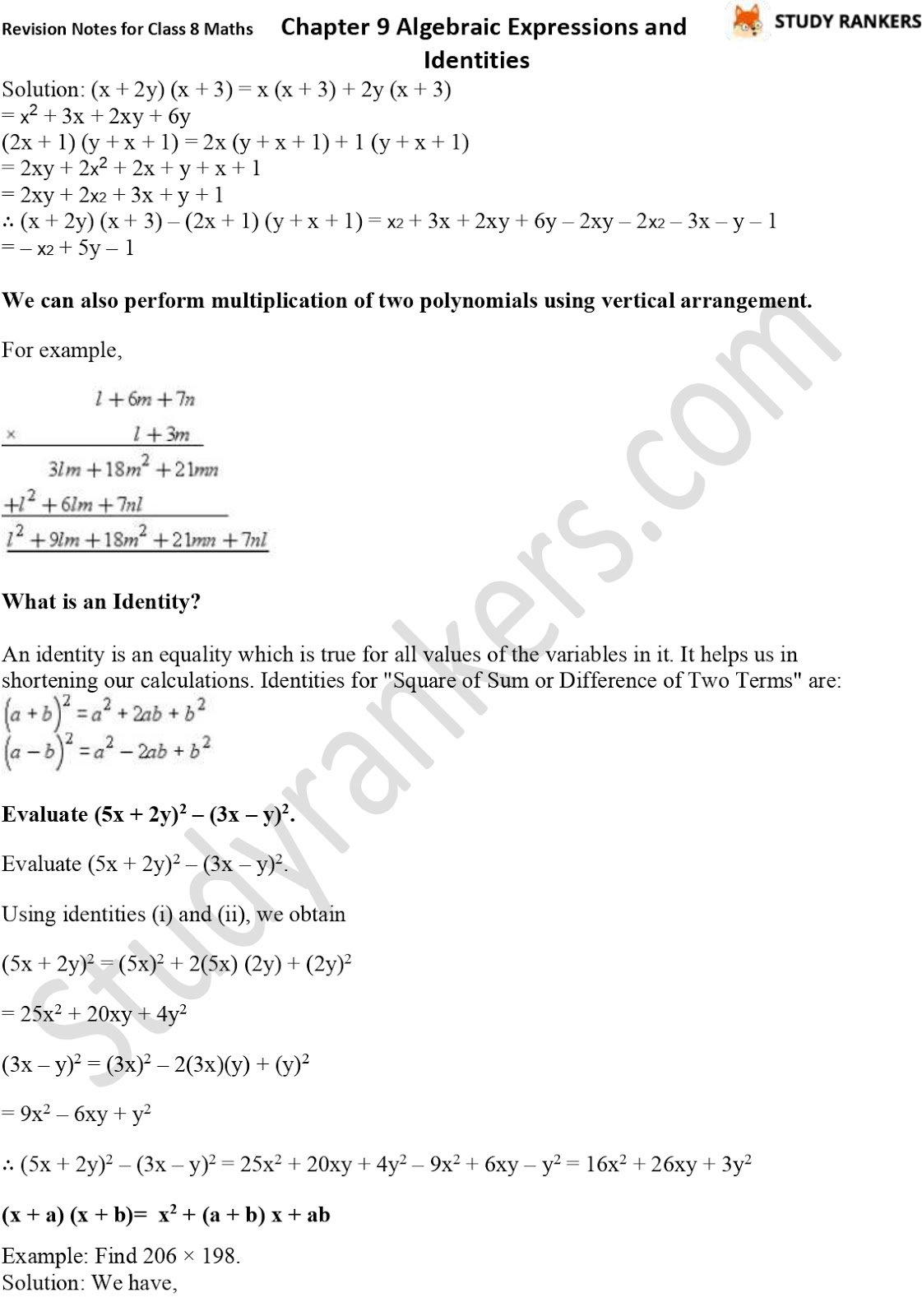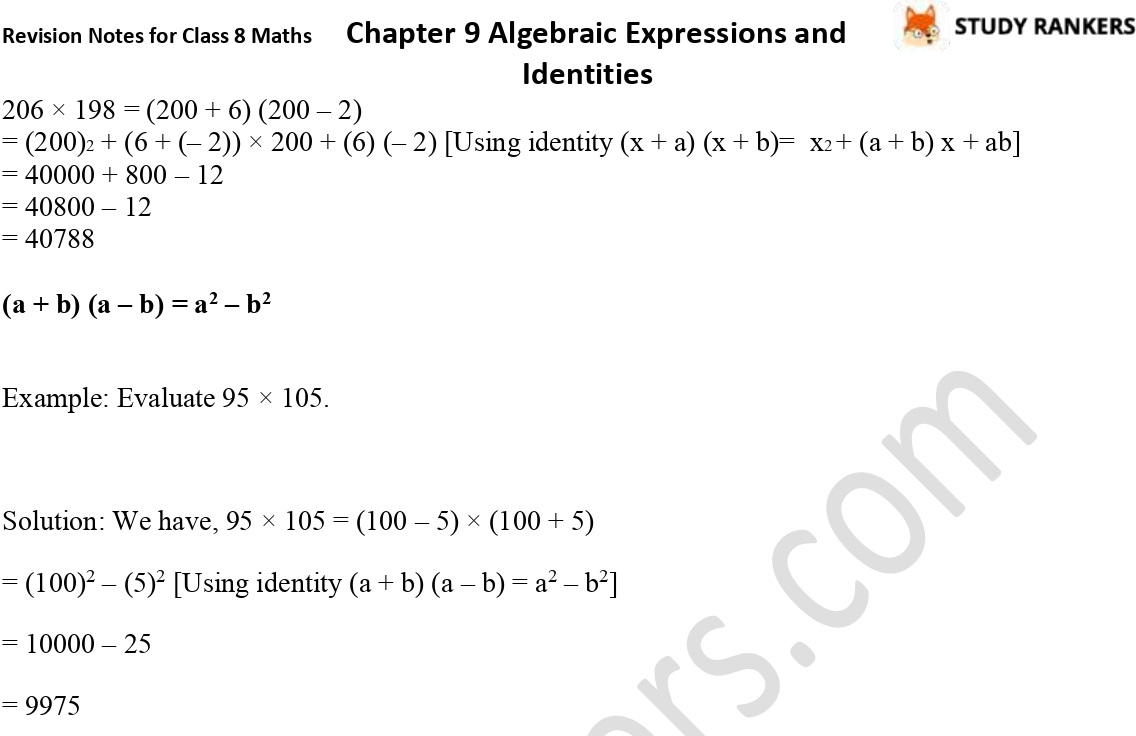>

CBSE Revision Notes for Class 8 Chapter 9 Algebraic Expressions and Identities

Chapter 9 Class 8 Revision Notes are very helpful if you want to complete chapter in less time. These Revision Notes for Class 8 Maths are prepared by Studyrankers subject matter experts who have large experience in teaching. These Maths Class 8 Notes will improve your knowledge and will useful in understanding concepts before examinations.

Topics in the chapter:
• What are Algebraic Expressions?
• Monomials, Binomials and Polynomials
• Like and Unlike Terms
• Addition and Subtraction of Algebraic Expressions
• Multiplication of monomial by a monomial
• Multiplication of a monomial by a binomial or trinomial or polynomial
• Multiplying a Polynomial by a Polynomial
• What is an Identity?
• Applying IdentitiesX